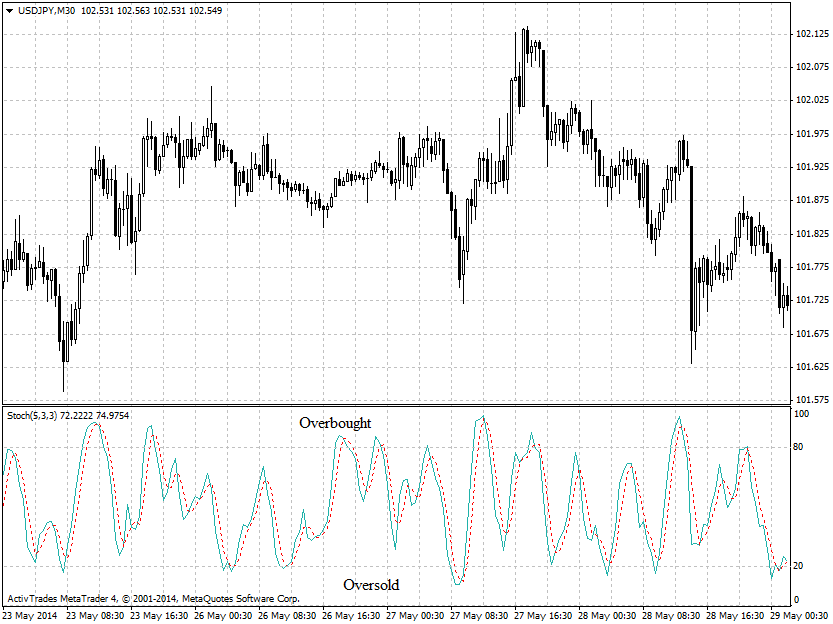# forex software

Create and Test Forex Strategies

forex software

trading:stochastic-oscillator

# Stochastic Oscillator

This indicator was developed by George Lane and shows the location of the close relative to the high-low of the price range over a set number of periods. This indicator attempts to predict market turning points by identifying overbought and oversold conditions.

The Stochastic Oscillator is calculated by the following formula

%K = 100 * (P - L(n))/(H(n)-L(n))

%D = SMA of %K,

where

P - current market price

L (n) - low for the last “n” periods

H (n) - high for the last “n” periods

Stochastic Oscillator is generating the following signals:

• Above 80 - overbought conditions
• Below 20 - oversold conditions
• %K and %D crosses
• Divergence of the price and indicator charts